前言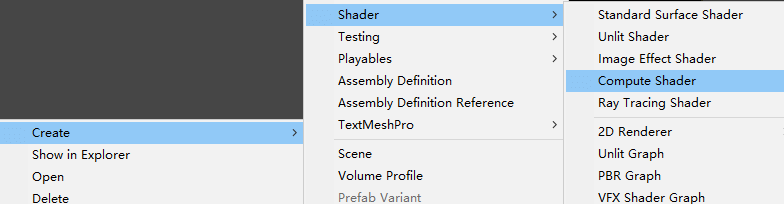#pragma kernel CSMain

RWTexture2D<float4> Result;

void CSMain (uint3 id : SV_DispatchThreadID)
{
Result[id.xy] = float4(id.x & id.y, (id.x & 15)/15.0, (id.y & 15)/15.0, 0.0);
}

语言

Unity使用的是DirectX 11的HLSL语言，会被自动编译到所对应的平台。

kernel

#pragma kernel CSMain

CSMain其实就是一个函数，在代码后面可以看到，而kernel是内核的意思，这一行即把一个名为CSMain的函数声明为内核，或者称之为核函数。这个核函数就是最终会在GPU中被执行。

#pragma kernel functionName

#pragma kernel KernelOne SOME_DEFINE DEFINE_WITH_VALUE=1337
#pragma kernel KernelTwo OTHER_DEFINE

#pragma kernel functionName // 一些注释

RWTexture2D

RWTexture2D<float4> Result;

RWTexture2D - Win32 apps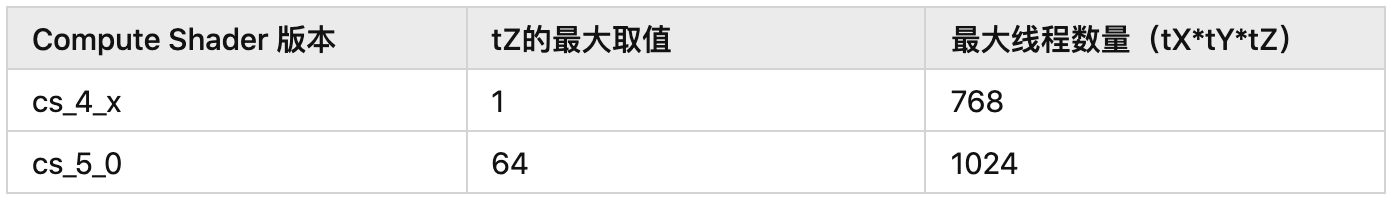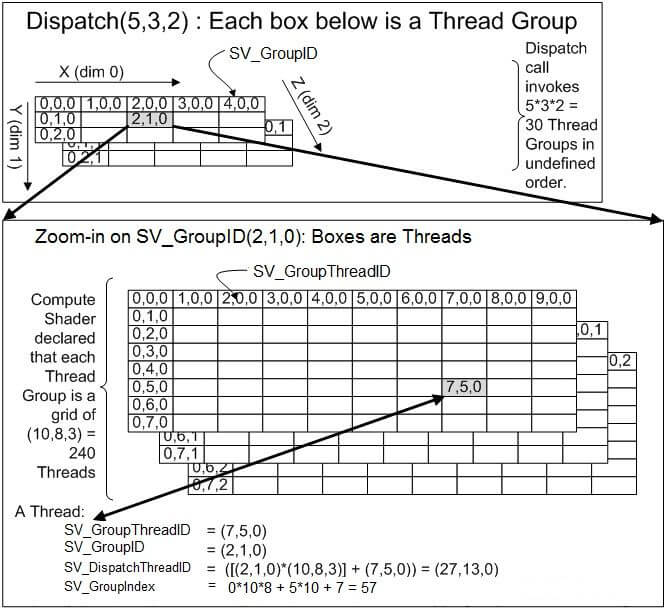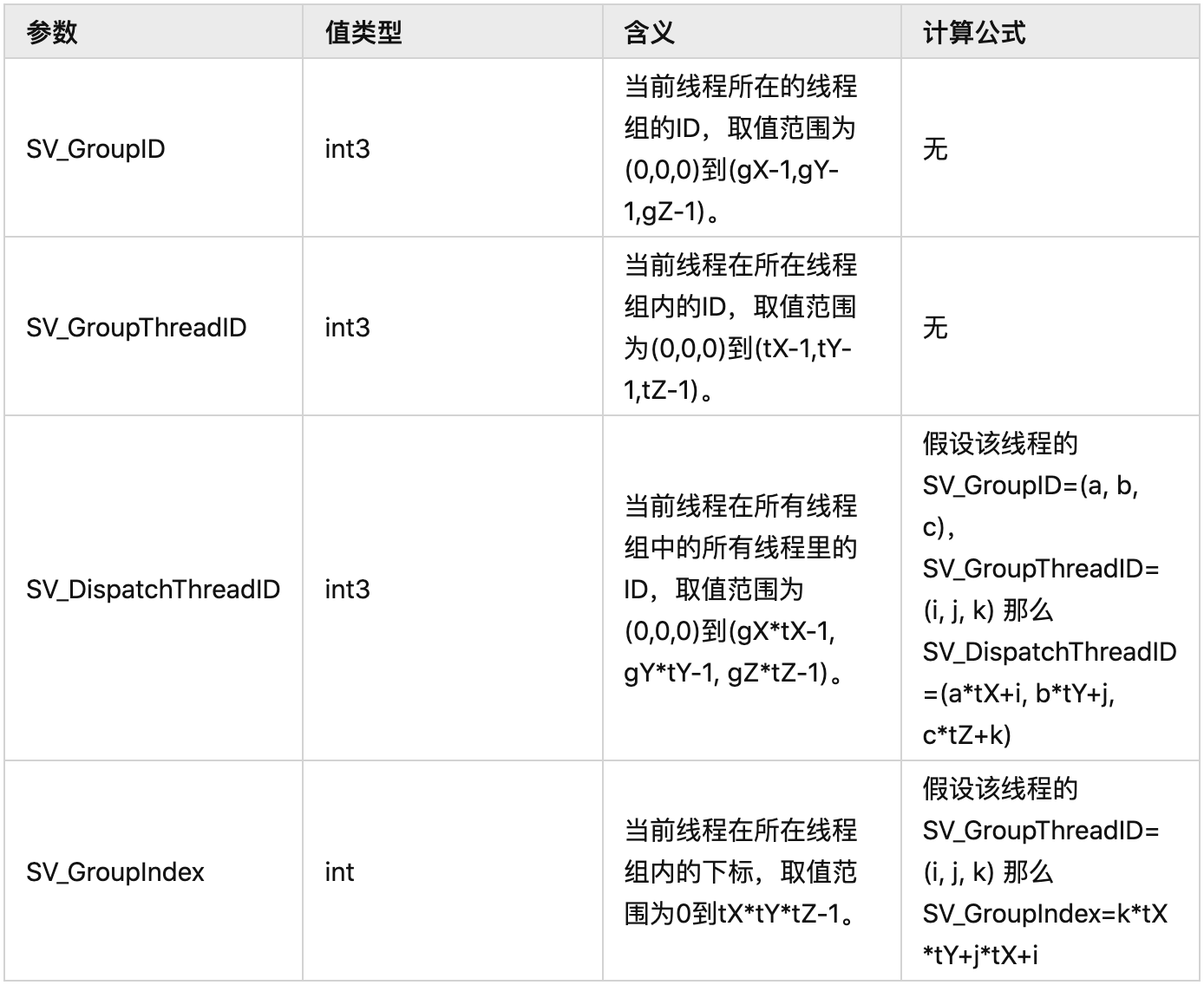核函数

void CSMain (uint3 id : SV_DispatchThreadID)
{
Result[id.xy] = float4(id.x & id.y, (id.x & 15)/15.0, (id.y & 15)/15.0, 0.0);
}

void KernelFunction(uint3 groupId : SV_GroupID,
uint groupIndex : SV_GroupIndex)
{

}

for (int i = 0; i < x; i++)
for (int j = 0; j < y; j++)
Result[uint2(x, y)] = float4(a, b, c, d);

{
for (int i = 0; i < x/4; i++)
for (int j = 0; j < y/4; j++)
Result[uint2(x, y)] = float4(a, b, c, d);
}

{
for (int i = x/4; i < x/2; i++)
for (int j = y/4; j < y/2; j++)
Result[uint2(x, y)] = float4(a, b, c, d);
}

{
for (int i = x/2; i < x/4*3; i++)
for (int j = x/2; j < y/4*3; j++)
Result[uint2(x, y)] = float4(a, b, c, d);
}

{
for (int i = x/4*3; i < x; i++)
for (int j = y/4*3; j < y; j++)
Result[uint2(x, y)] = float4(a, b, c, d);
}

{
for (int i = start; i < end; i++)
for (int j = start; j < end; j++)
Result[uint2(x, y)] = float4(a, b, c, d);
}

{
Result[uint2(x, y)] = float4(a, b, c, d);
}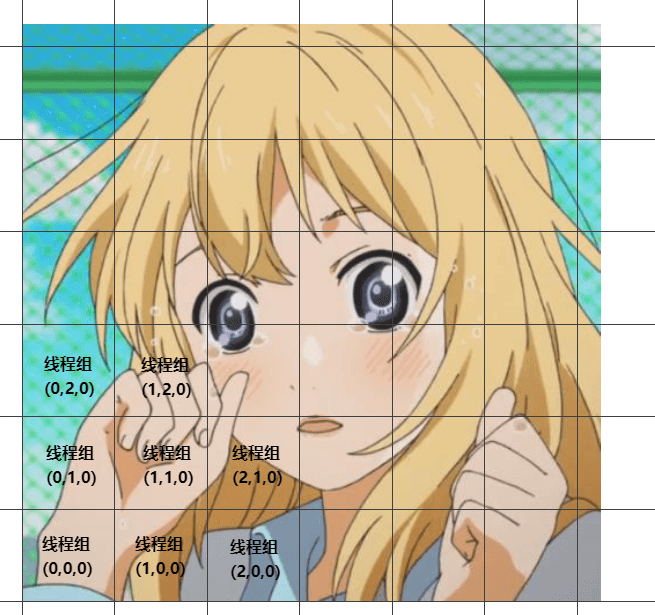C#部分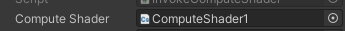public Material material;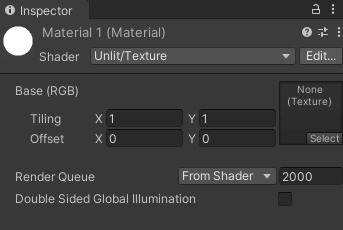RenderTexture mRenderTexture = new RenderTexture(256, 256, 16);
mRenderTexture.enableRandomWrite = true;
mRenderTexture.Create();

material.mainTexture = mRenderTexture;

fixed4 frag (v2f i) : SV_Target
{
// _MainTex 就是被处理后的 RenderTexture
fixed4 col = tex2D(_MainTex, i.uv);
return col;
}

computeShader.Dispatch(kernelIndex, 256 / 8, 256 / 8, 1);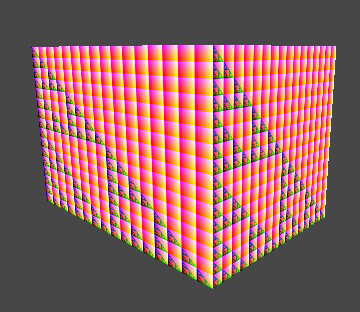struct ParticleData {
float3 pos;
float4 color;
};

RWStructuredBuffer

RWStructuredBuffer<ParticleData> ParticleBuffer;

RWStructuredBuffer - Win32 apps

float Time;

#pragma kernel UpdateParticle

struct ParticleData {
float3 pos;
float4 color;
};

RWStructuredBuffer<ParticleData> ParticleBuffer;

float Time;

void UpdateParticle(uint3 gid : SV_GroupID, uint index : SV_GroupIndex)
{
int pindex = gid.x * 1000 + index;

float x = sin(index);
float y = sin(index * 1.2f);
float3 forward = float3(x, y, -sqrt(1 - x * x - y * y));
ParticleBuffer[pindex].color = float4(forward.x, forward.y, cos(index) * 0.5f + 0.5, 1);
if (Time > gid.x)
ParticleBuffer[pindex].pos += forward * 0.005f;
}

ComputeBuffer

ComputeBuffer buffer = new ComputeBuffer(int count, int stride)

buffer.SetData(T[]);

public void SetBuffer(int kernelIndex, string name, ComputeBuffer buffer)

https://docs.unity3d.com/ScriptReference/ComputeBuffer.html

public struct ParticleData
{
public Vector3 pos;//等价于float3
public Color color;//等价于float4
}

void Start()
{
//struct中一共7个float，size=28
mParticleDataBuffer = new ComputeBuffer(mParticleCount, 28);
ParticleData[] particleDatas = new ParticleData[mParticleCount];
mParticleDataBuffer.SetData(particleDatas);
}

void Update()
{
}

{
{
Tags { "RenderType"="Opaque" }
LOD 100

Pass
{
CGPROGRAM
#pragma vertex vert
#pragma fragment frag

#include "UnityCG.cginc"

struct v2f
{
float4 col : COLOR0;
float4 vertex : SV_POSITION;
};

struct particleData
{
float3 pos;
float4 color;
};

StructuredBuffer<particleData> _particleDataBuffer;

v2f vert (uint id : SV_VertexID)
{
v2f o;
o.vertex = UnityObjectToClipPos(float4(_particleDataBuffer[id].pos, 0));
o.col = _particleDataBuffer[id].color;
return o;
}

fixed4 frag (v2f i) : SV_Target
{
return i.col;
}
ENDCG
}
}
}

public class ParticleEffect : MonoBehaviour
{
public Material material;

ComputeBuffer mParticleDataBuffer;
const int mParticleCount = 20000;
int kernelId;

struct ParticleData
{
public Vector3 pos;
public Color color;
}

void Start()
{
//struct中一共7个float，size=28
mParticleDataBuffer = new ComputeBuffer(mParticleCount, 28);
ParticleData[] particleDatas = new ParticleData[mParticleCount];
mParticleDataBuffer.SetData(particleDatas);
}

void Update()
{
material.SetBuffer("_particleDataBuffer", mParticleDataBuffer);
}

void OnRenderObject()
{
material.SetPass(0);
Graphics.DrawProceduralNow(MeshTopology.Points, mParticleCount);
}

void OnDestroy()
{
mParticleDataBuffer.Release();
mParticleDataBuffer = null;
}
}

OnRenderObject：该方法里我们可以自定义绘制几何。

DrawProceduralNow：我们可以用该方法绘制几何，第一个参数是拓扑结构，第二个参数数顶点数。

https://docs.unity3d.com/ScriptReference/Graphics.DrawProceduralNow.html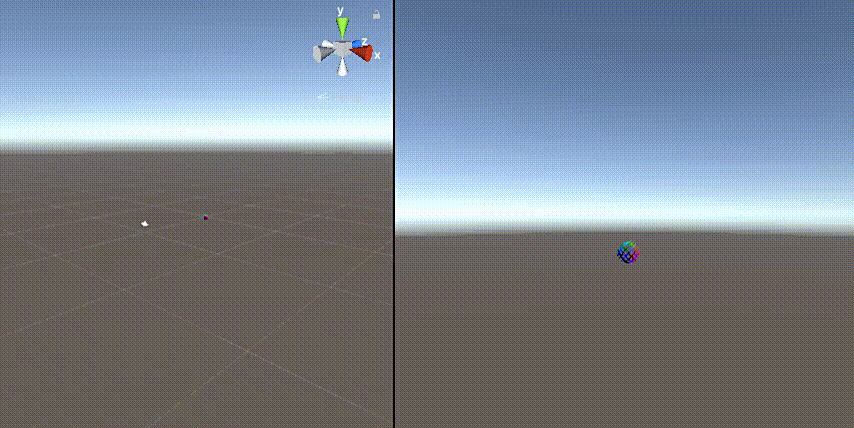ComputeBufferType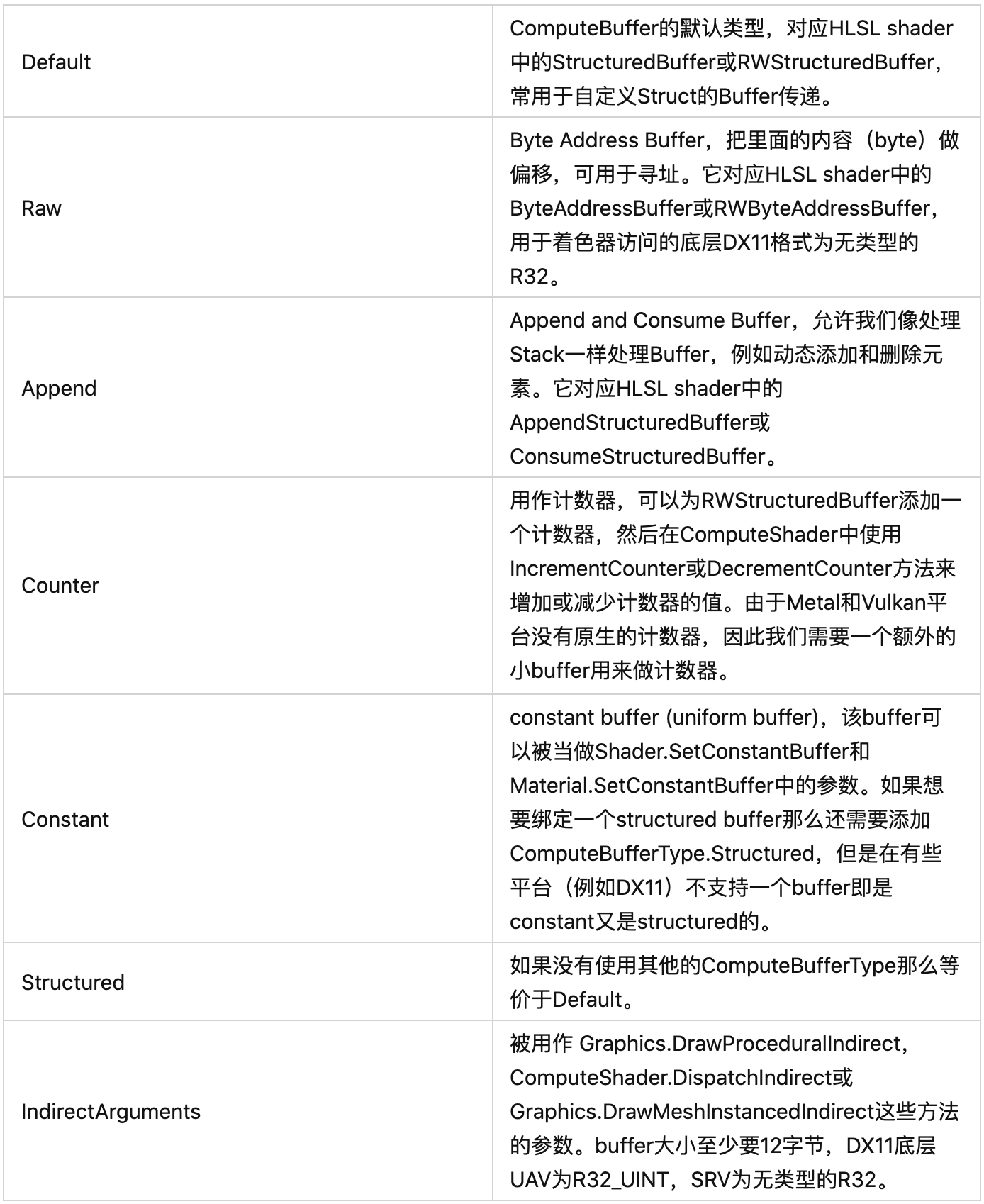var buffer = new ComputeBuffer(count, sizeof(float), ComputeBufferType.Append);

AppendStructuredBuffer<float> result;

{
if(满足一些自定义条件)
result.Append(value);
}

buffer.SetCounterValue(0);//计数器值为0

public static void CopyCount(ComputeBuffer src, ComputeBuffer dst, int dstOffsetBytes);

Append、Consume或者Counter的Buffer会维护一个计数器来存储Buffer中的元素数量，该方法可以把src中的计数器的值拷贝到dst中，dstOffsetBytes为在dst中的偏移。在DX11平台dst的类型必须为Raw或者IndirectArguments，而在其他平台可以是任意类型。

uint[] countBufferData = new uint { 0 };
var countBuffer = new ComputeBuffer(1, sizeof(uint), ComputeBufferType.IndirectArguments);
ComputeBuffer.CopyCount(buffer, countBuffer, 0);
countBuffer.GetData(countBufferData);
//buffer中的元素数量即为：countBufferData

UAV（Unordered Access view）

Unordered是无序的意思，Access即访问，view代表的是“data in the required format”，应该可以理解为数据所需要的格式吧。

the texture wasn't created with the UAV usage flag set!

Direct3D 12 术语表 - Win32 apps

Wrap / WaveFront

https://www.cvg.ethz.ch/teaching/2011spring/gpgpu/GPU-Optimization.pdf

移动端支持问题

#pragma target 4.5

int grassMatrixBufferId;
void Start() {
}
void Update() {
compute.SetBuffer(kernel, grassMatrixBufferId, grassMatrixBuffer);

// dont use it
//compute.SetBuffer(kernel, "grassMatrixBuffer", grassMatrixBuffer);
}

全局变量或常量？

#pragma kernel CSMain

float3 boxSize1 = float3(1.0f, 1.0f, 1.0f); // 方法1
const float3 boxSize2 = float3(2.0f, 2.0f, 2.0f); // 方法2
static float3 boxSize3 = float3(3.0f, 3.0f, 3.0f); // 方法3

void CSMain (uint3 id : SV_DispatchThreadID)
{
// 做判断
}

#pragma kernel CSMain
#pragma multi_compile __ COLOR_WHITE COLOR_BLACK

RWTexture2D<float4> Result;

void CSMain (uint3 id : SV_DispatchThreadID)
{
#if defined(COLOR_WHITE)
Result[id.xy] = float4(1.0, 1.0, 1.0, 1.0);
#elif defined(COLOR_BLACK)
Result[id.xy] = float4(0.0, 0.0, 0.0, 1.0);
#else
Result[id.xy] = float4(id.x & id.y, (id.x & 15) / 15.0, (id.y & 15) / 15.0, 0.0);
#endif
}

public class DrawParticle : MonoBehaviour
{